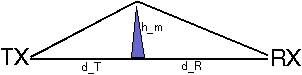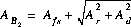### JPL's Wireless Communication Reference Website

#### Chapter: Wireless Propagation Channels Section: Path Loss# Diffraction lossFigure: Path profile model for (single) knife edge diffraction

If the direct line-of-sight is obstructed by a single knife-edge type of obstacle, with height hm we define the following diffraction parameter v:where dt and dR are the terminal distances from the knife edge. The diffraction loss, additional to free space loss and expressed in dB, can be closely approximated by
 AD=0 if v < 0 AD=6 + 9 v + 1.27 v2 if 0 < v < 2.4 AD=13 + 20 log v if v >2.4
The attenuation over rounded obstacles is usually higher than AD in the above formula.

### Input parameters:

Distance between transmitter and obstacle meter
Distance between receiver and obstacle meter
height of the obstacle meter
Frequency MHz

### Results:

Free space loss dB
SKE loss dB
Attenuation dB

Approximate techniques to compute the diffraction loss over multiple knife edges have been proposed by

• BullingtonFigure: Construction for approximate calculation of Multiple Knife-Edge diffraction loss, proposed by Bullington

The method by Bullington defines a new `effective' obstacle at the point where the line-of-sight from the two antennas cross.

• Epstein and PetersonFigure: Construction for approximate calculation of Multiple Knife-Edge diffraction loss, proposed by Epstein and Peterson

Epstein and Peterson suggested to draw lines-of-sight between relevant obstacles, and to add the diffraction losses at each obstacle.

• DeygoutFigure: Construction for approximate calculation of Multiple Knife-Edge diffraction loss, proposed by Deygout

Deygout suggested to search the `main' obstacle, i.e., the point with the highest value of v along the path. Diffraction losses over `secondary' obstacles are added to the diffraction loss over the main obstacle.

## Total Path loss

The previously presented methods for ground reflection loss and diffraction losses suggest a "Mondriaan" interpretation of the path profile: Obstacles occur as straight vertical lines while horizontal planes cause reflections. That is the propagation path is seen as a collection of horizontal and vertical elements. Accurate computation of the path loss over non-line-of-sight paths with ground reflections is a complicated task and does not allow such simplifications.

Many measurements of propagation losses for paths with combined diffraction and ground reflection losses indicate that knife edge type of obstacles significantly reduce ground wave losses. Blomquist suggested two methods to find the total loss:and the empirical formulawhere Afs the free space loss, AR the ground reflection loss and AD the multiple knife-edge diffraction loss in dB values.